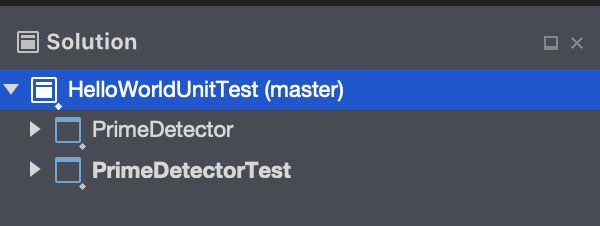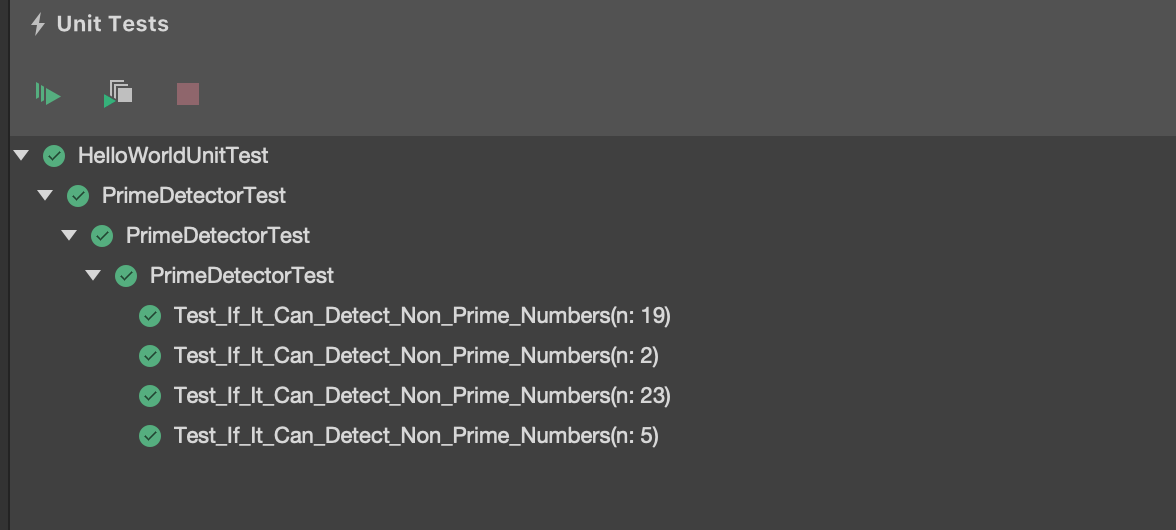# Introduction To Unit Test in .NET Core Applications By Xunit And Moq

Let’s assume, I have written some introductory sentences about what is unit testing and why it’s important. Let’s jump into main topic directly.

For writing unit test in .NET core applications we have some libraries like MSTest, NUnit , Xunit. In this post, I will be discussing about Xunit. For getting started, open your IDE and create a solution named HelloWorldUnitTest and add 2 projects, PrimeDetector and PrimeDetectorTestNow, we’ll write a function first, and then write unit test by using xunit. Lets start by writing the function, Lets add a class in PrimeDetector project named PrimeDetectorModel.cs

    public class PrimeDetectorModel
{
public bool IsPrime(int n)
{
var isPrime = true;

for (int i = 2; i < Math.Sqrt(n); i++)
{
if (n % i == 0) isPrime = false;
}

return isPrime;
}

}

Now we’ll write unit test for the isPrime()method. Let’s add a class named PrimeDetectorTest.cs in the PrimeDetectorTest project.

public class PrimeDetectorTest
{
[Fact]
public void Test_If_It_Can_Detect_A_Prime_Number()
{
// Arrange
var primeDetector = new PrimeDetectorModel();

// Act
var isPrime = primeDetector.IsPrime(29);

// Assert
Assert.Equal(isPrime, true);
}
}

What’s happening here?

With the [Fact]attribute, xUnit.net test runner identifies it’s a unit test to execute. Here, we will test whether our isPrime() identify a prime number or not. Every unit test has 3 parts:

1. Arrange: this portion will have code required to setup the test, in above example we need an instance of PrimeDetectorModel to call our isPrime() method.
2. Act: In this portion, we’ll call the function which we want to test. Eg: here we called IsPrime() with a prime number
3. Assert: this portion will responsible to judge whether our expectations were met or not. Eg: here, we checked value of isPrime is true or not.

How can we run this test multiple times with different input?

The above example tests whether our isPrime()method works for 29 or not. Now we want to test it for more integers, let’s say for 2, 5, 7, 19 and 23. Instead of copying and pasting the previous test function for 5 times, we can take advantage of [Theory] attribute. Our test function will now look like

	[Theory]
[InlineData(2)]
[InlineData(5)]
[InlineData(19)]
[InlineData(23)]
public void Test_If_It_Can_Detect_Non_Prime_Numbers(int n)
{

// Arrange
var primeDetector = new PrimeDetectorModel();

// Act
var isPrime = primeDetector.IsPrime(n);

// Assert
Assert.Equal(isPrime, true);
}

If we run this test, we will see our test function ran 4 times with the values we have given with [InlineData(n)] attribute.[Theory] attribute denotes a parameterised test, and by the help of the [InlineData] we provide the values for the parameter.

Summary: When we need to run the test once, we don’t need any parameter to be passed through the test function, we’ll use [Fact] attribute, otherwise we’ll use [Theory] attribute.

There are some limitations with [InlineData] attribute, it works with only constants. What if we need to send an object as a parameter?

Let’s add a PersonModel Class in PrimeDetector project.

 public class PersonModel
{
public int Id { get; set; }
public string Name { get; set; }
}

Now add HasPrimeId() method, bellow the isPrime() method. It will take an object of PersonModel and check whether the Id property is Prime or not.

        public bool HasPrimeId(PersonModel person)
{
return IsPrime(person.Id);
}

Now, we’ll write unit test for it. Go to the PrimeDetectorTest file and add this chunk of code.

    public static IEnumerable<object[]> GetTestData()
{
yield return new object[] { new PersonModel { Id = 5, Name = "Saadnoor" } };
yield return new object[] { new PersonModel { Id = 7, Name = "Salehin" } };
}

[Theory]
[MemberData(nameof(GetTestData))]
public void Test_If_It_Can_Detect_Person_Has_Prime_Id_Or_Not(PersonModel person)
{

// Arrange
var primeDetector = new PrimeDetectorModel();

// Act
var hasPrimeId = primeDetector.HasPrimeId(person);

// Assert
Assert.Equal(hasPrimeId, true);
}

It will call the test function Test_If_It_Can_Detect_Person_Has_Prime_Id_Or_Not() two times with 2 different PersonModel object.

1. { Id = 5, Name = "Saadnoor" }
2. { Id = 7, Name = "Salehin" } Note that, we have to set the return type of the GetTestData() method as IEnumerable<object[]> otherwise xUnit will throw an error. If we need 2 or more parameters in our test function, we can do that by adding it to the object[] array.

We can provide data with [ClassData] attribute also, but I’m skipping it in this post, to keep the post short and simple.

Now what if we have some dependencies to initiate our PrimeDetectorModel? How will we test it?

Moq will save us here. Let’s assume, to initiate PrimeDetectorModel we have a dependency of PositiveDetectorModel (which helps us to determine whether a number is positive or not).

Our PrimeDetectorModel class will now look like:

    public class PrimeDetectorModel
{
public PositiveDetector positiveDetector { get; set; }

public PrimeDetectorModel(PositiveDetector positiveDetector)
{
this.positiveDetector = positiveDetector;
}

public bool IsPrime(int n)
{
if (!positiveDetector.IsNumberPositive(n)) throw new Exception("Invalid Input");

var isPrime = true;

for (int i = 2; i < Math.Sqrt(n); i++)
{
if (n % i == 0) isPrime = false;
}

return isPrime;
}

public bool HasPrimeId(PersonModel person)
{
return IsPrime(person.Id);
}
}

Now our isPrime() function checks whether the given number is positive or not, if the number isn’t positive, it throws an Exception. It takes help from IsNumberPositive method, which comes from PositiveDetector model.

Now, while writing the test, we will create a mock of PositiveDetectorModel, and we are not bothered with isNumberPositive() method of this class, as we are writing unit test for isPrime() method, we’ll assume that, isNumberPositive works properly, we’ll mock it’s result too. Our test function is now looks like this.

        [Theory]
[InlineData(2)]
[InlineData(5)]
[InlineData(19)]
[InlineData(23)]
public void Test_If_It_Can_Detect_Non_Prime_Numbers(int n)
{
// Arrange
var positiveDetectorMock = new Mock<PositiveDetector>();

var primeDetector = new PrimeDetectorModel(
positiveDetectorMock.Object
);

positiveDetectorMock
.Setup(pd => pd.IsNumberPositive(n)).Returns(true);

// Act
var isPrime = primeDetector.IsPrime(n);

// Assert
Assert.Equal(isPrime, true);
}

Here, all the inputs (2, 5, 19, 23) are positive numbers, so we are mocking the isNumberPositive() function by returning true for these input.

 positiveDetectorMock
.Setup(pd => pd.IsNumberPositive(n)).Returns(true);

That’s pretty much covers the basic of writing unit tests with xUnit and Moq, for more details you can go to the resources of the reference section, unfortunately xUnit’s documentation sucks. Let me know if you have any feedback regarding this post by comments.

All my codes used here will be found here - https://github.com/saadnoor/UnitTestWithXunit

References:

Hey there, I am Saadnoor Salehin Shwapneel, a software engineer working at Cefalo AS, I

See more..